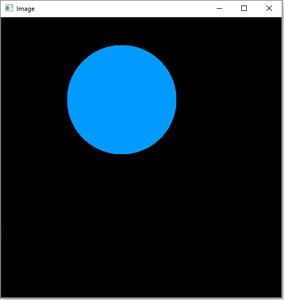Open In App
Related Articles

# Python OpenCV | cv2.circle() method

OpenCV-Python is a library of Python bindings designed to solve computer vision problems. cv2.circle() method is used to draw a circle on any image. The syntax of cv2.circle() method is:

Syntax:

`cv2.circle(image, center_coordinates, radius, color, thickness)`

Parameters:

• image: It is the image on which the circle is to be drawn.
• center_coordinates: It is the center coordinates of the circle. The coordinates are represented as tuples of two values i.e. (X coordinate value, Y coordinate value).
• color: It is the color of the borderline of a circle to be drawn. For BGR, we pass a tuple. eg: (255, 0, 0) for blue color.
• thickness: It is the thickness of the circle border line in px. Thickness of -1 px will fill the circle shape by the specified color.

Return Value: It returns an image.

The steps to create a circle on an image are:

2. Pass this image to the cv2.circle() method along with other parameters such as center_coordinates, radius, color and thickness.
3. Display the image using cv2.imshow() method.

Implementation:

Image used for all the below examples:Example #1:

## Python3

 `# Python program to explain cv2.circle() method ``   ` `# importing cv2 ``import` `cv2 ``   ` `# path ``path ``=` `r``'C:\Users\Rajnish\Desktop\geeksforgeeks\geeks.png'``   ` `# Reading an image in default mode``image ``=` `cv2.imread(path)``   ` `# Window name in which image is displayed``window_name ``=` `'Image'``  ` `# Center coordinates``center_coordinates ``=` `(``120``, ``50``)`` ` `# Radius of circle``radius ``=` `20``  ` `# Blue color in BGR``color ``=` `(``255``, ``0``, ``0``)``  ` `# Line thickness of 2 px``thickness ``=` `2``  ` `# Using cv2.circle() method``# Draw a circle with blue line borders of thickness of 2 px``image ``=` `cv2.circle(image, center_coordinates, radius, color, thickness)``  ` `# Displaying the image ``cv2.imshow(window_name, image) `

Output:Example #2: Using thickness of -1 px to fill the circle with red color.

## Python3

 `# Python program to explain cv2.circle() method ``   ` `# importing cv2 ``import` `cv2 ``   ` `# path ``path ``=` `r``'C:\Users\Rajnish\Desktop\geeksforgeeks\geeks.png'``   ` `# Reading an image in default mode``image ``=` `cv2.imread(path)``   ` `# Window name in which image is displayed``window_name ``=` `'Image'``  ` `# Center coordinates``center_coordinates ``=` `(``120``, ``100``)`` ` `# Radius of circle``radius ``=` `30``  ` `# Red color in BGR``color ``=` `(``0``, ``0``, ``255``)``  ` `# Line thickness of -1 px``thickness ``=` `-``1``  ` `# Using cv2.circle() method``# Draw a circle of red color of thickness -1 px``image ``=` `cv2.circle(image, center_coordinates, radius, color, thickness)``  ` `# Displaying the image ``cv2.imshow(window_name, image) `` `

Output:Example # 3: To display a circle on a black screen created using NumPy library.

## Python3

 `import` `cv2``import` `numpy as np``    ` ` ` `# Reading an image in default mode``Img ``=` `np.zeros((``512``, ``512``, ``3``), np.uint8)``    ` `# Window name in which image is displayed``window_name ``=` `'Image'``   ` `# Center coordinates``center_coordinates ``=` `(``220``, ``150``)``  ` `# Radius of circle``radius ``=` `100``   ` `# Red color in BGR``color ``=` `(``255``, ``133``, ``233``)``   ` `# Line thickness of -1 px``thickness ``=` `-``1``   ` `# Using cv2.circle() method``# Draw a circle of red color of thickness -1 px``image ``=` `cv2.circle(Img, center_coordinates, radius, color, thickness)``   ` `# Displaying the image``cv2.imshow(window_name, image)``cv2.waitKey(``0``)``cv2.destroyAllWindows()`

Output:Circle on Black background